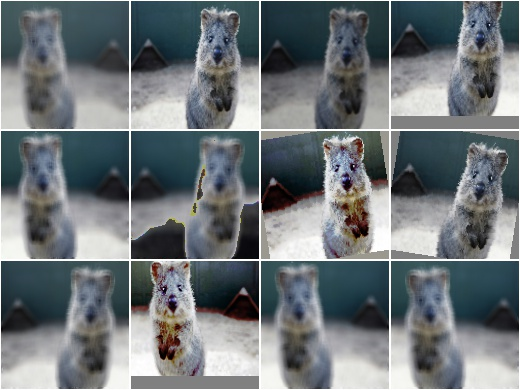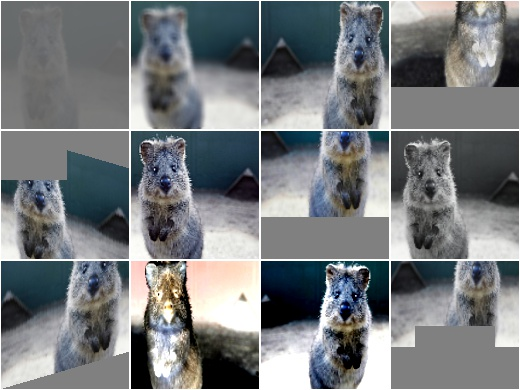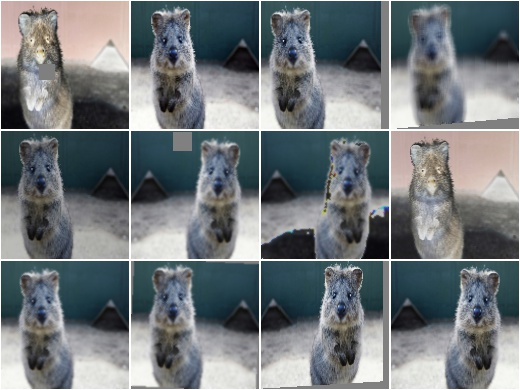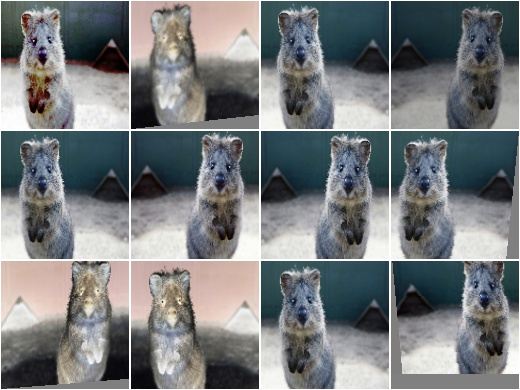# augmenters.collections¶

## RandAugment¶

Apply RandAugment to inputs as described in the corresponding paper.

See paper:

```Cubuk et al.

RandAugment: Practical automated data augmentation with a reduced
search space
```

Note

The paper contains essentially no hyperparameters for the individual augmentation techniques. The hyperparameters used here come mostly from the official code repository, which however seems to only contain code for CIFAR10 and SVHN, not for ImageNet. So some guesswork was involved and a few of the hyperparameters were also taken from https://github.com/ildoonet/pytorch-randaugment/blob/master/RandAugment/augmentations.py .

This implementation deviates from the code repository for all PIL enhance operations. In the repository these use a factor of `0.1 + M*1.8/M_max`, which would lead to a factor of `0.1` for the weakest `M` of `M=0`. For e.g. `Brightness` that would result in a basically black image. This definition is fine for AutoAugment (from where the code and hyperparameters are copied), which optimizes each transformation’s `M` individually, but not for RandAugment, which uses a single fixed `M`. We hence redefine these hyperparameters to `1.0 + S * M * 0.9/M_max`, where `S` is randomly either `1` or `-1`.

We also note that it is not entirely clear which transformations were used in the ImageNet experiments. The paper lists some transformations in Figure 2, but names others in the text too (e.g. crops, flips, cutout). While Figure 2 lists the Identity function, this transformation seems to not appear in the repository (and in fact, the function `randaugment(N, M)` doesn’t seem to exist in the repository either). So we also make a best guess here about what transformations might have been used.

Warning

This augmenter only works with image data, not e.g. bounding boxes. The used PIL-based affine transformations are not yet able to process non-image data. (This augmenter uses PIL-based affine transformations to ensure that outputs are as similar as possible to the paper’s implementation.)

API link: `RandAugment`

Example. Create a RandAugment augmenter similar to the suggested hyperparameters in the paper:

```import imgaug.augmenters as iaa
aug = iaa.RandAugment(n=2, m=9)
```Example. Create a RandAugment augmenter with maximum magnitude/strength:

```aug = iaa.RandAugment(m=30)
```Example. Create a RandAugment augmenter that applies its transformations with a random magnitude between `0` (very weak) and `9` (recommended for ImageNet and ResNet-50). `m` is sampled per transformation:

```aug = iaa.RandAugment(m=(0, 9))
```Example. Create a RandAugment augmenter that applies `0` to `3` of its child transformations to images. Horizontal flips (p=50%) and crops are always applied.

```aug = iaa.RandAugment(n=(0, 3))
```